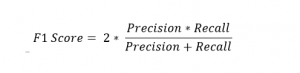Blogs/F1 Score

# F1 Score

blazedafyah Jul 22 2021 1 min read 173 views

NIT Warangal graduate, Software Engineer at Wipro.

FundamentalsSeveral metrics can be used to evaluate the performance of a binary classifier. Accuracy is the simplest of all and is defined as the ratio of correctly classified examples divided by the total number of examples.

Accuracy is a widely-used and straightforward metric that is easy to implement. However, it doesn’t take into account cases where there is a large class imbalance (more than 90% of examples belonging to one class) or cases with different costs for false positives and false negatives.

Precision and recall are two complementary metrics that evaluate the classifier’s correctness. Precision is a measure of relevant data points or simply a ratio between true positives and all the positives. The recall is the ratio of correctly predicted positive observations to all observations in that class or simply the ratio between true positives and the sum of true positives and false negatives.

While both precision and recall mitigate the weakness of accuracy, there is a tradeoff involved in evaluating the model on these two. Improving one comes at the cost of the other. There are situations where one of them can be prioritized, while in others, both precision and recall are equally important.

In the latter case, the F1 score is used as an evaluation metric. It is the harmonic mean of Precision and Recall.A good F1 score would be indicative of good precision and recall, and now we only have to work with one metric. It is simply conveying the balance between both.

Learn and practice this concept here:

https://mlpro.io/problems/f1-score/